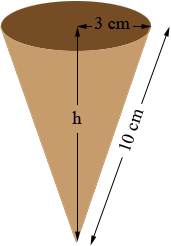SEARCH HOMEMath Central Quandaries & QueriesQuestion from Tamriko, a student: Hi! Help me, please to solve the following problem: The diameter of an ice-cream cone is 6 cm and the slant height is 10 cm. What volume of ice-cream would fit inside the cone? Thanks!Tamriko,

The volume of a cone is 1/3 π r2 h where r is the radius of the open end of the cone and h is the height. You know the radius is 6/2 = 3 cm but you know the slant height rather than the height.What does Pythagoras theorem tell you about the height?

PennyMath Central is supported by the University of Regina and The Pacific Institute for the Mathematical Sciences.